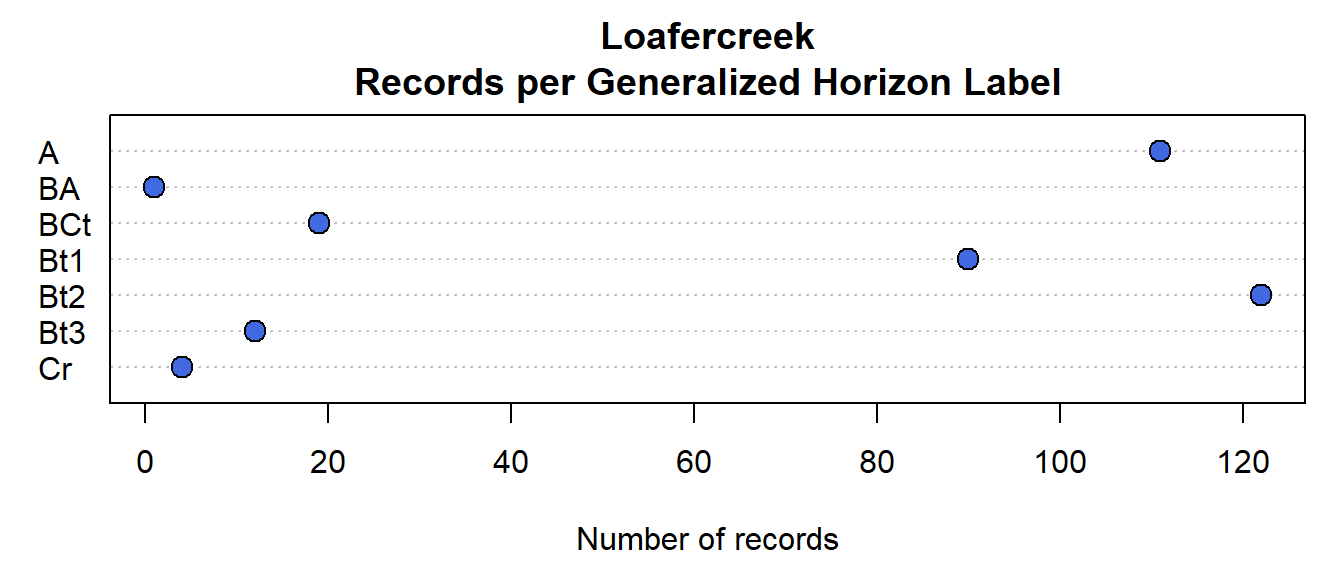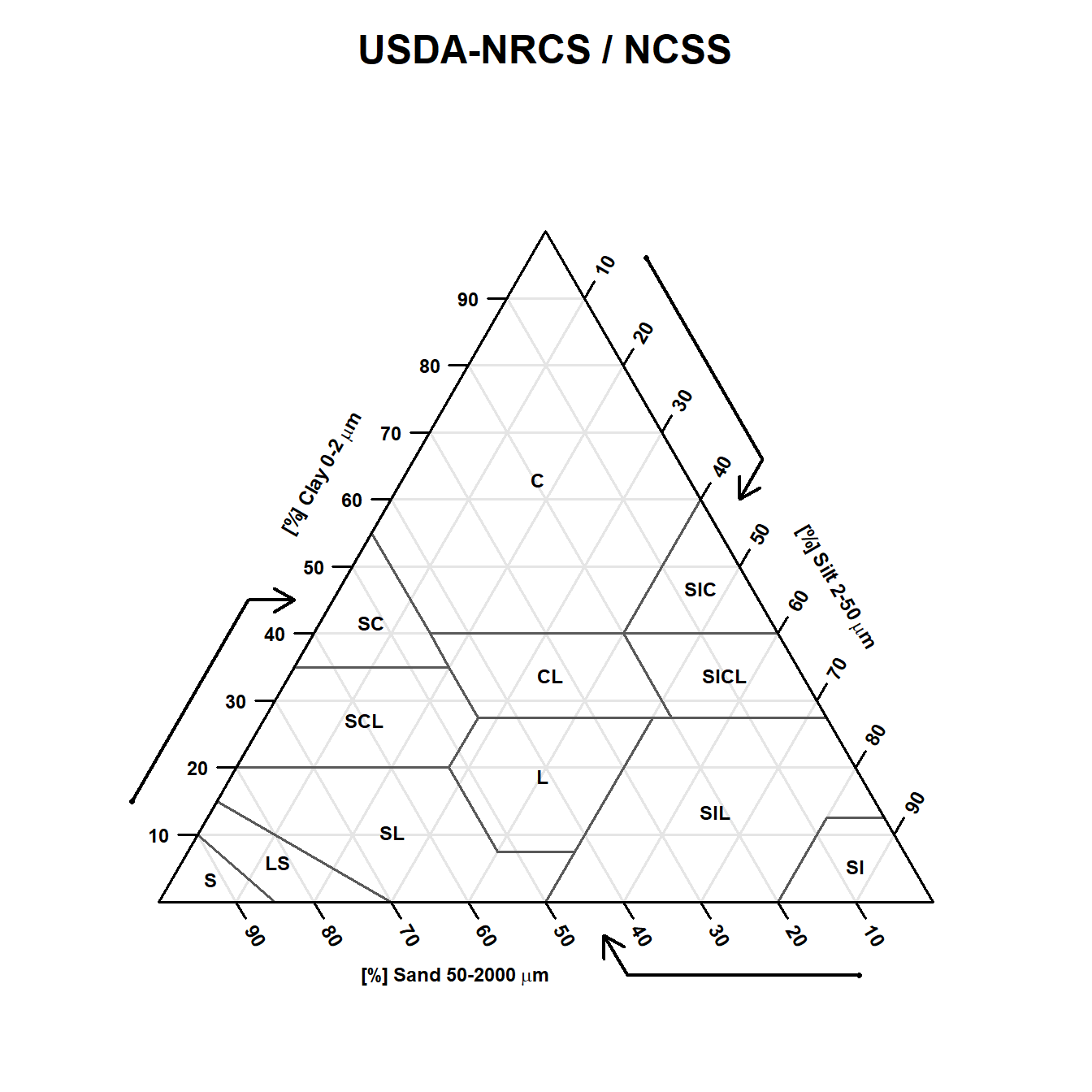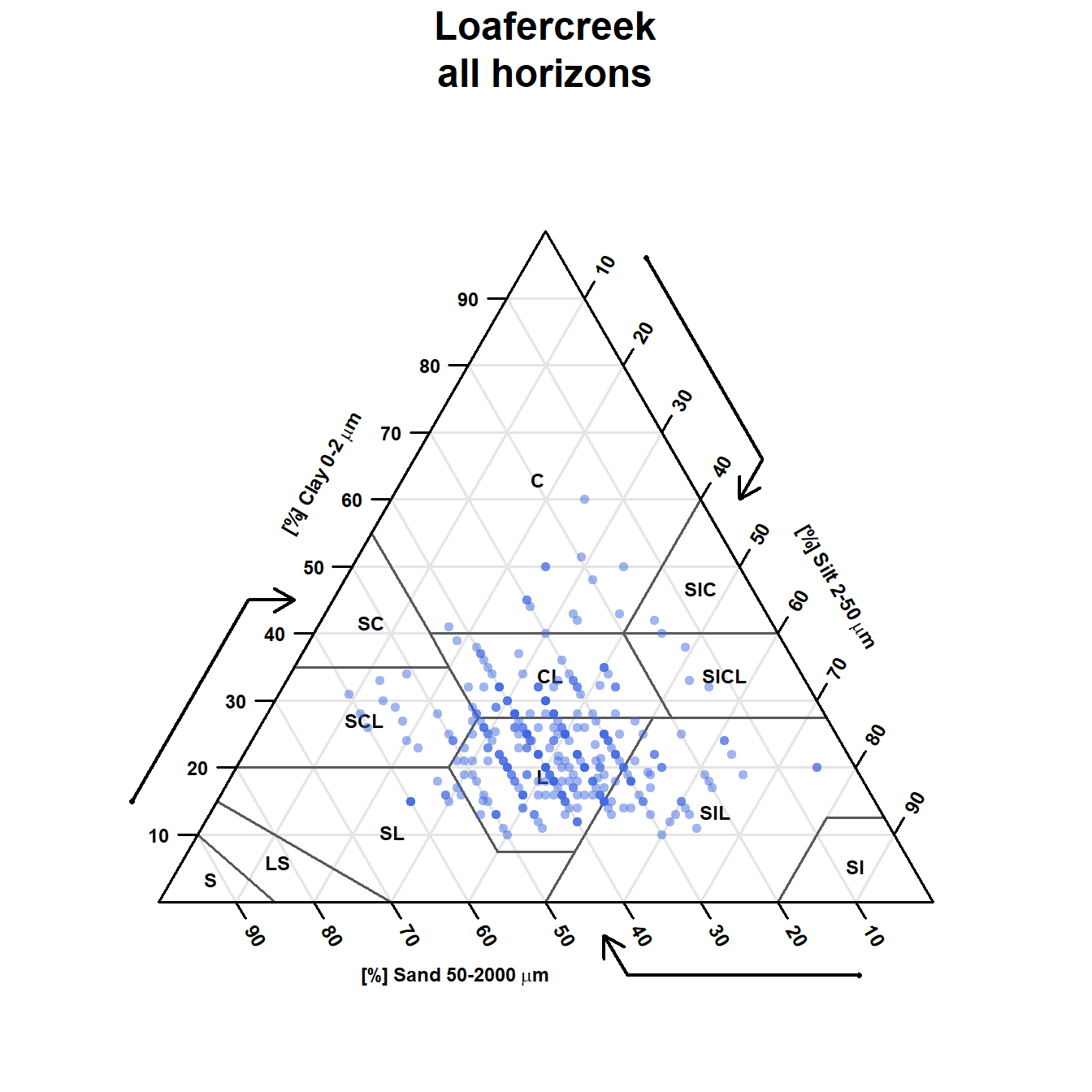# 1 Examples with the Loafercreek sample data

Load required packages, sample data, and subset of `horizon` attributes.

``````# SoilProfileCollection methods
# https://github.com/ncss-tech/aqp/
library(aqp)
# data-getting / sample data
library(soilDB)
# soil texture triangle
library(soiltexture)
# convenient alpha() function
library(scales)

# load sample data from soilDB package
data("loafercreek")

# extract just horizon data
h <- horizons(loafercreek)``````

Subset `sand`, `silt`, `clay` percentages and filter missing records or inconsistent fractions (e.g. add to more or less than 100%). Column names are adjusted to accommodate subsequent usage.

``````# extract components of field texture, removing rows with missing data
ssc <- h[, c('genhz', 'sand', 'silt', 'clay')]
ssc <- na.omit(ssc)

# adjust names for plotting with TT.plot()
# names must be SAND, SILT, CLAY
names(ssc) <- toupper(names(ssc))

# test of bogus data
ssc\$sum <- rowSums(ssc[, c('SAND', 'SILT', 'CLAY')])
# > 5% deviation from 100%
idx <- which(abs(ssc\$sum - 100) > 5)

# check errors
ssc[idx, ]``````
``````##     GENHZ SAND SILT CLAY sum
## 623     A   45   62   17 124``````
``````# ommit errors
# maybe fix these later?
ssc <- ssc[-idx, ]

# check: OK
``````##    GENHZ SAND SILT CLAY sum
## 23     A   40   48   12 100
## 24   Bt1   40   48   12 100
## 25   Bt1   45   41   18 104
## 26   Bt2   50   32   18 100
## 27   BCt   50   34   16 100
## 29     A   15   66   19 100``````
``````# split by generalized horizon name into a list of data.frames
ssc.list <- split(ssc, ssc\$GENHZ)

record.count <- rev(sapply(ssc.list, nrow))

par(mar = c(4.5, 3, 3, 1))
dotchart(record.count, pch = 21, bg = 'royalblue', pt.cex = 1.5, xlab = 'Number of records', main = 'Loafercreek\nRecords per Generalized Horizon Label')``````# 2 Visualization via `soiltexture` Package

An empty soil texture triangle, based particle size classes and abbreviations used by the US. Department of Agriculture, Natural Resources Conservation Service (USDA-NRCS) and National Cooperative Soil Survey (NCSS). This interface to ternary diagrams is provided by the excellent `soiltexture` package by Julien Moeys.

``````TT.plot(
class.sys = "USDA-NCSS.TT",    # use "our" texture triangle
main = 'USDA-NRCS / NCSS',          # title
cex.lab = 0.75,                 # scaling of label text
cex.axis = 0.75,                # scaling of axis
frame.bg.col = 'white',         # background color
class.lab.col = 'black',        # color for texture class labels
lwd.axis = 1.5,
lwd.lab = 2,
arrows.show=TRUE
)``````Create a new figure, this time saving the ternary diagram geometry for later (`TT` object). Data can be added as needed with the `TT.` family of functions.

``````# init figure and save geometry
TT <- soiltexture::TT.plot(
class.sys = "USDA-NCSS.TT",    # use "our" texture triangle
main = 'Loafercreek\nall horizons',          # title
tri.sum.tst = FALSE,            # do not test for exact sum(sand, silt, clay) == 100
cex.lab = 0.75,                 # scaling of label text
cex.axis = 0.75,                # scaling of axis
frame.bg.col = 'white',         # background color
class.lab.col = 'black',        # color for texture class labels
lwd.axis = 1.5,
lwd.lab = 2,
arrows.show=TRUE
)

# screen coordinates are available for later use
xy <- TT.points(tri.data = ssc, geo = TT, tri.sum.tst = FALSE, cex=0.5, col=alpha('royalblue', 0.5))````````head(xy)``
``````##   xpos     ypos
## 1 54.0 10.39230
## 2 54.0 10.39230
## 3 46.0 15.58846
## 4 41.0 15.58846
## 5 42.0 13.85641
## 6 75.5 16.45448``````

Subset data to `A` horizons, all with one call to `TT.plot`.

``````# local copy of `A` horizons
x <- ssc.list\$A

# compose title using the current genhz
this.hz <- x\$GENHZ
title.text <- sprintf("Loafercreek: %s Horizons", this.hz)

TT.plot(
class.sys= "USDA-NCSS.TT",    # use "our" texture triangle
tri.data=x,                 #
main=title.text,    # title
tri.sum.tst=FALSE,            # do not test for exact sum(sand, silt, clay) == 100
cex.lab=0.75,                 # scaling of label text
cex.axis=0.75,                # scaling of axis
cex=0.5,                      # scaling of point symbols
col=alpha('darkgreen', 0.5),  # color of point symbols, with 50% transparency
frame.bg.col='white',         # background color
class.lab.col='black',        # color for texture class labels
lwd.axis=2                    # line thickness for axis
)``````